The regression equation for change in temperature, y, to amount of rain, r, is given by ŷ = 0.2 − 1.4r. On Monday, the observed amount of ra

Question

The regression equation for change in temperature, y, to amount of rain, r, is given by ŷ = 0.2 − 1.4r. On Monday, the observed amount of rain was 0.4 inches and the temperature change was 2.3 degrees. Find and interpret the residual.

2.66. The regression line overpredicts the temperature change.
2.66. The regression line underpredicts the temperature change.
1.94. The regression line underpredicts the temperature change.
−1.94. The regression line overpredicts the temperature change.
−3.02. The regression line underpredicts the temperature change.

in progress 0
5 months 2021-08-22T11:14:11+00:00 1 Answers 8 views 0

1. Given:

The regression equation for change in temperature, y, to amount of rain, r, is given by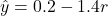The observed amount of rain was 0.4 inches and the temperature change was 2.3 degrees.

To find:

The residual and interpret it.

Solution:

According to the question, the observed amount of rain was 0.4 inches and the temperature change was 2.3 degrees.

So, the actual value is 2.3 degrees.

Put r=0.4 in the given equation, to find the predicted value.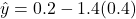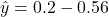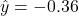We know that,

Residual = Actual value – Predicted value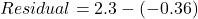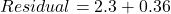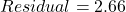Residual is positive because predicated value is less than the actual value.

So, the regression line underpredicts the temperature change.

Therefore, the correct option is B.